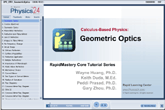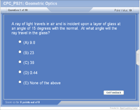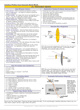How to Learn in 24 Hours?The Rapid Learning Movie

 Need Help? M-F: 9am-5pm(PST): Toll-Free: (877) RAPID-10 US Direct: (714) 692-2900 Int'l: 001-714-692-2900 24/7 Online Technical Support: The Rapid Support Center Secure Online Order:Got Questions? Frequently Asked Questions
 Need Proof? Testimonials by Our Users
 Trustlink is a Better Business Bureau Program. Rapid Learning Center is a fivr-star business. External TrustLink Reviews

 Rapid Learning Courses: MCAT in 24 Hours (2015-16) USMLE in 24 Hours (Boards) Chemistry in 24 Hours Biology in 24 Hours Physics in 24 Hours Mathematics in 24 Hours Psychology in 24 Hours SAT in 24 Hours ACT in 24 Hours AP in 24 Hours CLEP in 24 Hours DAT in 24 Hours (Dental) OAT in 24 Hours (Optometry) PCAT in 24 Hours (Pharmacy) Nursing Entrance Exams Certification in 24 Hours eBook - Survival Kits Audiobooks (MP3)

 Tell-A-Friend: Have friends taking science and math courses too? Tell them about our rapid learning system.Home »  Physics »  Calculus Physics

Geometric Optics

 Topic Review on "Title": Law of reflection: The angle of incidence equals the angle of reflection. Diffuse reflection: Reflection from a rough surface where variations in the direction of the surface cause light to reflect in different directions. Convex mirror: Also called a diverging mirror, a surface that diverges light as if it originates from a point behind the mirror, a focal point. Concave mirror: Also, called a converging mirror, a surface that converges light to a single point, focal point. Real image: An image where the rays of light actually meet at a location.  It can be projected onto a screen. Virtual image: An image that cannot be projected onto a screen.  The rays of light don’t actually converge there, they just seem to originate from that location. Refraction: The bending of light due to its change in velocity in various media. Index of refraction: The ratio between the speed of light in a vacuum and a particular medium. Snell’s law: The formula that describes the amount of refraction of light based on the two different media and the angle of the light ray:  n1sinθ1 = n2sinθ2 Total internal reflection: The complete reflection of light when it strikes the boundary between two media at greater than a critical angle. Convex lens: A converging lens that gathers incoming light to a single focal point. Concave lens: A diverging lens that diverges light as if it originates from a point behing the lens, the focal point. Spherical aberration: A lens defect where light is imperfectly focused near the focal point. Chromatic aberration: A lens defect where various color focus at different locations.

Rapid Study Kit for "Title":
 Flash Movie Flash Game Flash Card Core Concept Tutorial Problem Solving Drill Review Cheat Sheet"Title" Tutorial Summary : Light can reflect as it bounces off a sufficiently smooth barrier.  In doing this, it will obey the law of reflection that says the incident angle is equal to the reflected angle.  Reflection can also occur for curved mirrors, both convex and concave.  In these instances, real or virtual images can be formed.  Ray diagrams can be used to trace the behavior of light and determine the image.  The mirror formula can also be used to calculate variables.  When light passes into transparent materials, it may refract or bend.  Snell’s law describes this quantitatively.  Sometimes the light is bend so much that it doesn’t emerge from the transparent material.  This is called total internal reflection.  This bending of light is the basic behind the operation of a lens.  Convex and concave lenses can form real and virtual images too.  Again, both ray diagrams and the lens equation can be used to describe the resulting image.

 Tutorial Features: Specific Tutorial Features: • Many animated ray diagrams for mirrors and lenses showing image formation. • Examples with step by step assistance to solve problems. Series Features: • Concept map showing inter-connections of new concepts in this tutorial and those previously introduced. • Definition slides introduce terms as they are needed. • Visual representation of concepts • Animated examples—worked out step by step • A concise summary is given at the conclusion of the tutorial.

 "Title" Topic List: Plane mirrors Law of reflection Virtual images Diffuse reflection Importance or wavelength Curved mirrors Concave mirror ray diagram Convex mirror ray diagram Mirror equation and example Magnification equation and example Refraction Index of refraction Refraction example and analogy Snell’s law Dispersion Total internal reflection Fiber optics Lenses Convex lens ray diagram Concave lens ray diagram Lens equation and example Lens combinations Lens defects

See all 24 lessons in high school  physics, including concept tutorials, problem drills and cheat sheets:
Teach Yourself Calculus Physics Visually in 24 Hours